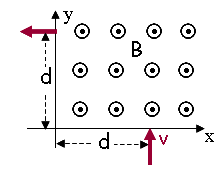# Problem: A charged particle of mass m = 5.6×10-8 kg, moving with constant velocity in the y-direction enters a region containing a constant magnetic field B = 3.5 T aligned with the positive z-axis as shown. The particle enters the region at (x,y) = (0.83 m, 0) and leaves the region at (x,y) = 0, 0.83 m a time t = 776 μs after it entered the region.1) With what speed v did the particle enter the region containing the magnetic field? (m/s)2) What is Fx, the x-component of the force on the particle at a time t1 = 258.7 μs after it entered the region containing the magnetic field? (N)3) What is Fy, the y-component of the force on the particle at a time t1 = 258.7 μs after it entered the region containing the magnetic field? (N)4) What is q, the charge of the particle? Be sure to include the correct sign. (μC)5) If the velocity of the incident charged particle were doubled, how would B have to change (keeping all other parameters constant) to keep the trajectory of the particle the same?A. Increase B by a factor of 2B. Increase B by less than a factor of 2C. Decrease B by less than a factor of 2D. Decrease B by a factor of 2E. There is no change that can be made to B to keep the trajectory the same.

###### FREE Expert Solution

1)

Speed, v = d/t

d = 2πR/4

Substituting:

92% (22 ratings)###### Problem DetailsA charged particle of mass m = 5.6×10-8 kg, moving with constant velocity in the y-direction enters a region containing a constant magnetic field B = 3.5 T aligned with the positive z-axis as shown. The particle enters the region at (x,y) = (0.83 m, 0) and leaves the region at (x,y) = 0, 0.83 m a time t = 776 μs after it entered the region.

1) With what speed v did the particle enter the region containing the magnetic field? (m/s)

2) What is Fx, the x-component of the force on the particle at a time t1 = 258.7 μs after it entered the region containing the magnetic field? (N)

3) What is Fy, the y-component of the force on the particle at a time t1 = 258.7 μs after it entered the region containing the magnetic field? (N)

4) What is q, the charge of the particle? Be sure to include the correct sign. (μC)

5) If the velocity of the incident charged particle were doubled, how would B have to change (keeping all other parameters constant) to keep the trajectory of the particle the same?

A. Increase B by a factor of 2

B. Increase B by less than a factor of 2

C. Decrease B by less than a factor of 2

D. Decrease B by a factor of 2

E. There is no change that can be made to B to keep the trajectory the same.